# Scale of the map

The distance between two cities is actually 30 km and the map is 6 cm. What is the scale of the map?

M =  500000

### Step-by-step explanation: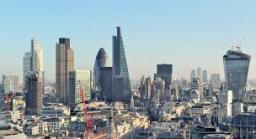Did you find an error or inaccuracy? Feel free to write us. Thank you!Tips to related online calculators
Need help to calculate sum, simplify or multiply fractions? Try our fraction calculator.
Check out our ratio calculator.
Do you want to convert length units?

## Related math problems and questions:

• Scale of the mapDetermine the map's scale, which is the actual distance of 120 km l represented by a segment long 6 cm.
• Map scale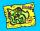What is the scale of the map, if 5.4 km is 72 mm on the map?
• The distance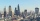The distance between two towns on a given map is 3 ½ cm. If ½ cm represents 6km. , what is the distance between the two towns?
• Map 2At what scale is made map if the distance 8.2 km corresponds on the map segment 5 cm long?
• Two monuments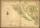The distance between two historical monuments on a map with a scale of 1: 500000 is 48 mm. Find the actual distance between these monuments in km.
• Two citiesOn a map, 1.5 cm represents 50 miles. If the distance between the two cities on the map is 6 cm, how many miles separate the cities?
• Two villages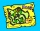On the map with a scale of 1:40000 are drawn two villages actually 16 km away. What is their distance on the map?
• The scaleThe distance of 15 cm on the map corresponds to a distance of 27 km in reality. What is the scale of the map?
• Map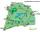On the tourist map at a scale of 1 : 50000 is distance between two points along a straight road 3.7 cm. How long travels this distance on a bike at 30 km/h? Time express in minutes.
• On the mapA line 1.5 cm long corresponds to a line 3 cm long on the map. What is the scale of the map?
• What scale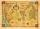What scale is the map drawn if it shows a 15km route from the station to the ruins with a line 30cm long? A) 1: 2000 B) 1: 5000 C) 1: 20,000 D) 1: 50,000
• Scale of the mapDetermine the scale of the map if the actual distance between A and B is 720 km and the distance on the map is 20 cm.
• Small city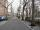The length of the street is 230 m. What will be the length of its image on the plan at a scale of 1: 2500?
• Two villages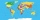Two villages are 11 km and 500 m away. On the map, their distance is determined by a 5 cm long line. Find the scale of the map.
• The plan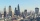The plan of the housing estate is in three scales 1: 5000,1: 10000,1: 15000. The distance between two points on a plan with a scale of 1: 10000 is 12 cm. What is this distance on the other two plans? What is this distance?
• Map 3Map scale is M = 1: 25000 . Two cottages which are shown on the map are actually 15 km away. What is its distance on the map?
• The sidewalk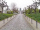The sidewalk is 50 m long. What will be its length on the plot plan on a scale of 1: 200?